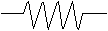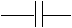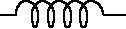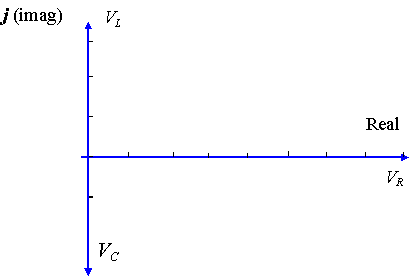Search IntMath
Close

450+ Math Lessons written by Math Professors and Teachers

5 Million+ Students Helped Each Year

1200+ Articles Written by Math Educators and Enthusiasts

Simplifying and Teaching Math for Over 23 Years

# 8. An Application of Complex Numbers: AC Circuits

by M. Bourne

Before we see how complex numbers can help us to analyse and design AC circuits, we first need to define some terms.

### Resource

You can find more background on this material at Electronics Tutorials

## Definitions

### Resistance

Symbol: R

Units: Ω (ohms)

A resistor is any part of a circuit that obstructs the flow of current.### Capacitance

Symbol: C

Units: "F" (farads)

A capacitor consists of 2 non-connected plates:Continues below

### Inductance

Symbol: L

Units: "H" (henrys)

An inductor is coil of wire in which current is induced.Current: I (in amperes)

Voltage: V (in volts).

Ohm's Law: V = IR

### Reactance

Reactance is the effective resistance of any part of the circuit. This could be from an inductor or a capacitor. See more in the next section Impedance and Phase Angle.

Symbol: X

## Voltage in alternating current circuits

• The voltage across a resistance is in phase with the current .

• The voltage across a capacitor lags the current by 90^@.

• The voltage across an inductance leads the current by 90^@

For voltage: V = IX

The voltage across a resistor with resistance R:

VR = IRThe voltage across a capacitor with reactance XC (voltage and current are RMS, or 'root mean square' values):

VC = IXCThe voltage across an inductor with reactance XL (once again, voltage and current are RMS values):

VL = IXL## Representing voltages using the complex plane

Using the complex plane, we can represent voltages across resistors, capacitors and inductors.

The voltage across the resistor is regarded as a real quantity, while the voltage across an inductor is regarded as a positive imaginary quantity, and across a capacitor we have a negative imaginary quantity. Our axes are as follows:## Problem SolverThis tool combines the power of mathematical computation engine that excels at solving mathematical formulas with the power of GPT large language models to parse and generate natural language. This creates math problem solver thats more accurate than ChatGPT, more flexible than a calculator, and faster answers than a human tutor. Learn More.